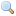# Module Queue

`module Queue: `sig` .. `end``
First-in first-out queues.

This module implements queues (FIFOs), with in-place modification.

`type `'a` t `
The type of queues containing elements of type `'a`.
`exception Empty`
Raised when `Queue.take` or `Queue.peek` is applied to an empty queue.
`val create : `unit -> 'a t``
Return a new queue, initially empty.
`val add : `'a -> 'a t -> unit``
`add x q` adds the element `x` at the end of the queue `q`.
`val push : `'a -> 'a t -> unit``
`push` is a synonym for `add`.
`val take : `'a t -> 'a``
`take q` removes and returns the first element in queue `q`, or raises `Empty` if the queue is empty.
`val pop : `'a t -> 'a``
`pop` is a synonym for `take`.
`val peek : `'a t -> 'a``
`peek q` returns the first element in queue `q`, without removing it from the queue, or raises `Empty` if the queue is empty.
`val top : `'a t -> 'a``
`top` is a synonym for `peek`.
`val clear : `'a t -> unit``
Discard all elements from a queue.
`val copy : `'a t -> 'a t``
Return a copy of the given queue.
`val is_empty : `'a t -> bool``
Return `true` if the given queue is empty, `false` otherwise.
`val length : `'a t -> int``
Return the number of elements in a queue.
`val iter : `('a -> unit) -> 'a t -> unit``
`iter f q` applies `f` in turn to all elements of `q`, from the least recently entered to the most recently entered. The queue itself is unchanged.
`val fold : `('b -> 'a -> 'b) -> 'b -> 'a t -> 'b``
`fold f accu q` is equivalent to `List.fold_left f accu l`, where `l` is the list of `q`'s elements. The queue remains unchanged.
`val transfer : `'a t -> 'a t -> unit``
`transfer q1 q2` adds all of `q1`'s elements at the end of the queue `q2`, then clears `q1`. It is equivalent to the sequence `iter (fun x -> add x q2) q1; clear q1`, but runs in constant time.